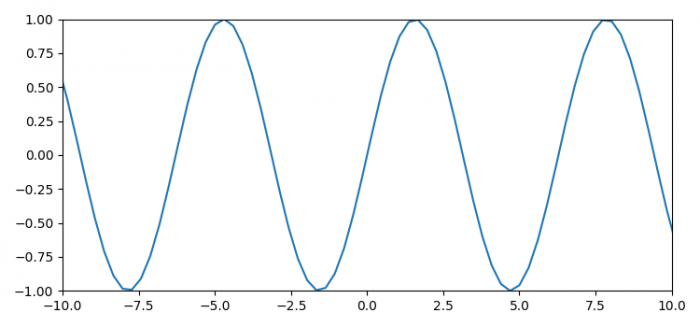# How to change the range of the X-axis and Y-axis in Matplotlib?

To change the range of X and Y axes, we can use xlim() and ylim() methods.

## Steps

• Set the figure size and adjust the padding between and around the subplots.
• Create x and y data points using numpy.
• Plot x and y data points using plot() method.
• Set the X and Y axes limit.
• To display the figure, use show() method.

## Example

import numpy as np
import matplotlib.pyplot as plt

plt.rcParams["figure.figsize"] = [7.50, 3.50]
plt.rcParams["figure.autolayout"] = True

x = np.linspace(-15, 15, 100)
y = np.sin(x)

plt.plot(x, y)

plt.xlim(-10, 10)
plt.ylim(-1, 1)

plt.show()

## Output